# SAT Part 1 — Boolean logic

This is the first part of the SAT series. For your convenience you can find other parts using the links below:
SAT Part 1 — Boolean logic
SAT Part 2 — Arithmetic
Part 3 — Reducing ILP to SAT

Today we are going to implement some Boolean logic in SAT.

# Equal and not equal

Let’s begin with equality. We have a clause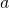and we want to add clause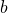which is equal to. This means that ifisin some assignment thenisas well. We get this with the following:

Ifisthen first clause is satisfied. For the second to be satisfiedmust beas well.
However, ifis zero then second clause is satisfied but the first one is not. In that case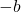must besomust be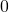.

For inequality (or binary negation) we have the following:

Whenisthen we need to haveset toin second clause sois. Whenisthenmust bein the first clause.

# Conjunction

We have clausesand, and we want to have clause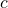being conjunction ofand:

For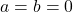we have last clause makebeing.
For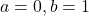third clause makesbeing.
For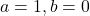we have second clause doing the same.
Finally, for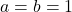we have first clause which makesequal to.

# Disjunction

Goes this way:

Forwe have first clause makingequal to. All other clauses cover other permutations.

# XOR

For exclusive or we can use:

Forthird clause makes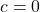. Forfourth clause does the same. Similarly, for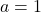and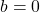we have first clause which makes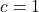.

# Introducing numbers to the model

Let’s now say that we would like to introduce some constant integer to the model. What we need to do is represent it in U2 system and set flags accordingly.

Let’s take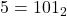. We add one clause for each bit with the following constraints:

You can see that this will correctly assign values to clauses and from now on we can use these clauses as bits of the number.

In the next part we will implement some arithmetic.